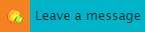## What is the GRE Quantitative section about?

The GRE Quantitative section, also known as the Quantitative Reasoning (QR) section, essentially tests your ability to deal with facts and figures. Don’t worry, it’s not all complex number crunching – your ability to arrive at a solution in a logical and stepwise manner is just as important. Many questions are “word problems” which must be logically modelled mathematically.

## What is the format of the Quantitative Reasoning section?

There are two (or three, depending on the experimental section) 35 minute sections, each containing 20 questions. Enough said.

## What are some of the topics that show up in this section?

Some important topics covered include:

• Integral calculus
• Game Theory
• Partial Differential Equations

Just kidding. (I’m sorry!)

The GRE QR section contains topics you probably studied in high school – stuff like properties of numbers, basic geometric shapes, algebra, word problems, data interpretation and so on.

You can safely assume the following about numbers and figures in the Quantitative Reasoning section:

• All numbers used are real numbers
• Geometric figures, such as lines, circles, triangles and quadrilaterals, are not necessarily drawn to scale
• You should assume that all geometric objects are in the relative positions shown. For these questions, do not on estimate quantities by sight or by measurement
• Coordinate systems, such as xy-planes and number lines, are drawn to scale; so, you can estimate quantities in such figures by sight or by measurement
• Graphical data presentations, such as bar graphs, circle graphs, and line graphs, are drawn to scale

The Official Guide to the GRE Revised General Test provides an insight into the topics likely to appear on the GRE’s Quantitative Section. Going through this is very highly recommended.

## What are the types of questions that are asked?

According to the ETS’ official website, the GRE Quantitative Reasoning section comprises of 4 types of questions:

### Quantitative Comparison questions

Usually seven or eight in number, these questions appear at the beginning of each section. Every question contains 2 columns – each of which evaluates to a value. The two values then need to be compared and the option which correctly represents the relationship between the two values is the right answer.

### Multiple choice questions with ONE correct answer

As the name suggests, every question contains five answer choices – one of which is the right one.

### Multiple choice questions with ONE or more correct answer

Such questions require you to select one or more answer choices from the list of choices provided. The choices are represented by square boxes rather than the regular ovals. The right answer is either a single option or a combination of options. Note that in order to score full points in such a question, all correct options must be chosen and all incorrect options must not be selected. There are no part points for such questions.

### Numeric Entry questions

These type of questions do not provide any answer choices – the test taker is expected to solve the question and write the answer, in integer or decimal format, in the box provided. In case of fractional answers, enter the answer in two separate boxes – one each for the numerator and the denominator.

All questions are not of the same difficulty – some are harder than the others. You’re likely to lose more points if you incorrectly answer an easier question than a more difficult one!

So that brings us to the big question:

## How do I ace this section?

Don’t worry, you don’t need to be a “math” person to do well in this section. This section tests your ability to grasp basic (I mean very basic) mathematical concepts and apply these to arrive at the right answer. With some practice (and maybe a teeny weeny bit of luck), you too can master the math section!

In the next article, I elaborate on some of the tips, tricks and hacks which helped me ace the Quantitative Reasoning section. Stay tuned for more!